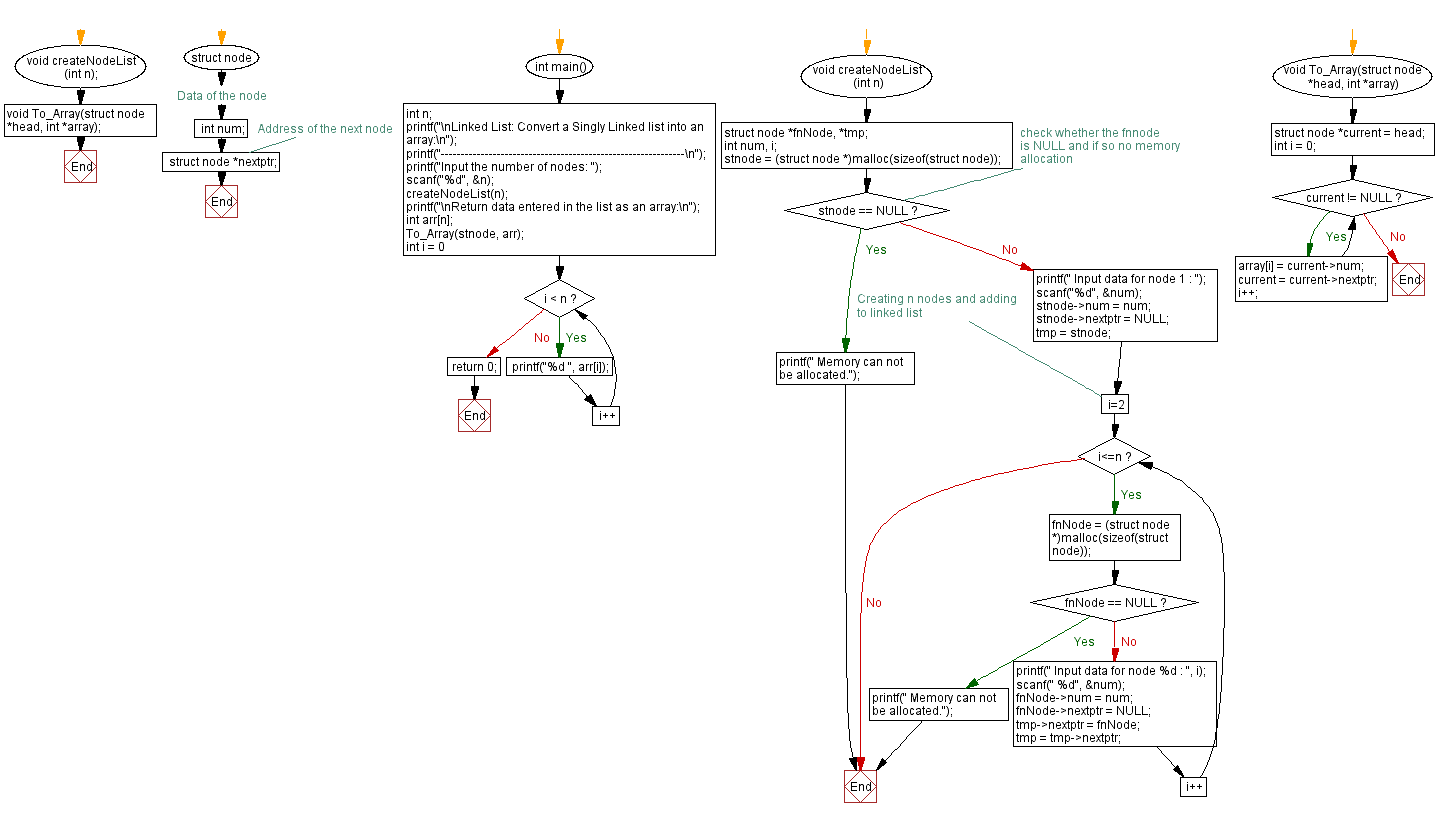﻿ C Program: Convert a Singly Linked list into a array

# C Exercises: Convert a Singly Linked list into a array

## C Singly Linked List : Exercise-12 with Solution

Write a C program that converts a singly linked list into an array and returns it.

Sample Solution:

C Code:

``````#include<stdio.h>
#include <stdlib.h>
#include <string.h>

struct node
{
int num;                         //Data of the node
struct node *nextptr;   //Address of the next node
}*stnode;

void createNodeList(int n);
void To_Array(struct node *head, int *array);

int main()
{
int n;
printf("-------------------------------------------------------------\n");
printf("Input the number of nodes: ");
scanf("%d", &n);
createNodeList(n);
printf("\nReturn data entered in the list as an array:\n");
int arr[n];
To_Array(stnode, arr);
for (int i = 0; i < n; i++)
printf("%d ", arr[i]);
return 0;
}

void createNodeList(int n)
{
struct node *fnNode, *tmp;
int num, i;
stnode = (struct node *)malloc(sizeof(struct node));

if(stnode == NULL) //check whether the fnnode is NULL and if so no memory allocation
{
printf(" Memory can not be allocated.");
}
else
{
printf(" Input data for node 1 : ");
scanf("%d", &num);
stnode->num = num;
stnode->nextptr = NULL;
tmp = stnode;
for(i=2; i<=n; i++)
{
fnNode = (struct node *)malloc(sizeof(struct node));
if(fnNode == NULL)
{
printf(" Memory can not be allocated.");
break;
}
else
{
printf(" Input data for node %d : ", i);
scanf(" %d", &num);

fnNode->num = num;
fnNode->nextptr = NULL;

tmp->nextptr = fnNode;
tmp = tmp->nextptr;
}
}
}
}
void To_Array(struct node *head, int *array) {
int i = 0;
while (current != NULL) {
array[i] = current->num;
current = current->nextptr;
i++;
}
}
```
```

Sample Output:

```
-------------------------------------------------------------
Input the number of nodes: 4
Input data for node 1 : 10
Input data for node 2 : 20
Input data for node 3 : 30
Input data for node 4 : 40

Return data entered in the list as an array:
10 20 30 40
```

Flowchart :C Programming Code Editor:

What is the difficulty level of this exercise?

﻿